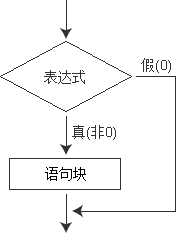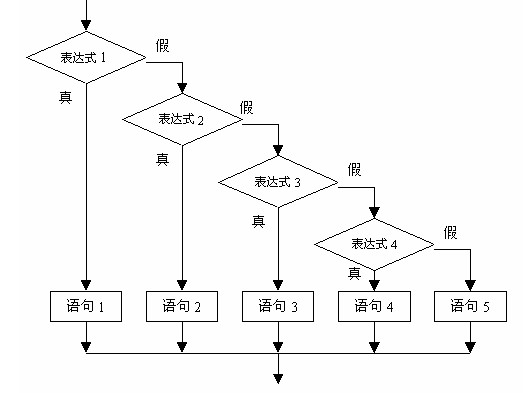# C语言 if else 语句详细讲解if-else语句

```#include
int main()
{
int age;
printf("请输入你的年龄：");
scanf("%d", &age);
if(age>=18){
printf("恭喜，你已经成年，可以使用该软件！\n");
}else{
printf("抱歉，你还未成年，不宜使用该软件！\n");
}
return 0;
}
```

if else 语句的结构为：

if(表达式){

}else{

}if(age>=18) printf(“恭喜，你已经成年，可以使用该软件！\n”);
else printf(“抱歉，你还未成年，不宜使用该软件！\n”);

```#include
int main()
{
int a, b, max;
printf("输入两个整数：");
scanf("%d %d", &a, &b);
if(a>b) max=a;
else max=b;
printf("%d和%d的较大值是：%d\n", a, b, max);
return 0;
}
```

34和28的较大值是：34

## if语句

if(表达式){

}```#include
int main()
{
int a, b, max;
printf("输入两个整数：");
scanf("%d %d", &a, &b);
max=b; // 假设b最大
if(a>b) max=a; // 如果a>b，那么更改max的值
printf("%d和%d的较大值是：%d\n", a, b, max);
return 0;
}
```

34和28的较大值是：34

## 多个if else语句

if else语句也可以多个同时使用，构成多个分支，形式如下：

if(表达式1){

} else  if(表达式2){

}else  if(表达式3){

}else  if(表达式m){

}else{

}```#include
int main(){
char c;
printf("Input a character:");
c=getchar();
if(c<32)
printf("This is a control character\n");
else if(c>='0'&&c<='9')
printf("This is a digit\n");
else if(c>='A'&&c<='Z')
printf("This is a capital letter\n");
else if(c>='a'&&c<='z')
printf("This is a small letter\n");
else
printf("This is an other character\n");
return 0;
}
```

Input a character:e
This is a small letter

1) 在三种形式的if语句中，在 if 关键字之后均为表达式。 该表达式通常是逻辑表达式或关系表达式，但也可以是其它表达式，如赋值表达式等，甚至也可以是一个变量。例如：
if(a=5) 语句;
if(b) 语句;

if(a=5)…;

```if(a=b)
printf("%d",a);
else
printf("a=0");
```

2) 在if语句中，条件判断表达式必须用括号括起来。

3) 语句块由{ }包围，但要注意的是在}之后不要再加分号;。例如：

```if(a>b){
a++;
b++;
}else{
a=0;
b=10;
}
```

## if语句的嵌套

if 语句也可以嵌套使用，例如：

```#include
int main(){
int a,b;
printf("Input two numbers:");
scanf("%d %d",&a,&b);
if(a!=b){
if(a>b) printf("a>b\n");
else printf("a<b\n");
}else{
printf("a=b\n");
}
return 0;
}
```

Input two numbers:12 68
a<b

if 语句嵌套时，要注意 if 和 else 的配对问题。C语言规定，else 总是与它前面最近的 if 配对，例如：

```if(a!=b) // ①
if(a>b) printf("a>b\n"); // ②
else printf("a<b\n"); // ③
```

③和②配对，而不是和①配对。